# NCERT Solutions for Class 7 Maths Chapter 10 – Practical Geometry

Class 7 Maths NCERT Solutions Chapter 10 – Practical Geometry comprises of the 5 Exercises

This Chapter contains the Exercises relating to the following topics , which are discussed in Chapter 10 – Practical Geometry Class 7 NCERT book  : –

• 10.1 INTRODUCTION
• 10.2 CONSTRUCTION OF A LINE PARALLEL TO A GIVEN LINE, THROUGH A POINT NOT ON THE LINE
• 10.3 CONSTRUCTION OF TRIANGLES
• 10.4 CONSTRUCTING A TRIANGLE WHEN THE LENGTHS OF ITS THREE SIDES ARE KNOWN (SSS CRITERION)
• 10.5 CONSTRUCTING A TRIANGLE WHEN THE LENGTHS OF TWO SIDES AND THE MEASURE OF THE ANGLE BETWEEN THEM ARE KNOWN. (SAS CRITERION)
• 10.6 CONSTRUCTING A TRIANGLE WHEN THE MEASURES OF TWO OF ITS ANGLES AND THE LENGTH OF THE SIDE INCLUDED BETWEEN THEM IS GIVEN. (ASA CRITERION)
• 10.7 CONSTRUCTING A RIGHT-ANGLED TRIANGLE WHEN THE LENGTH OF ONE LEG AND ITS HYPOTENUSE ARE GIVEN (RHS CRITERION)

### NCERT Solutions for Class 7 Maths Chapter 10 Exercise 10.1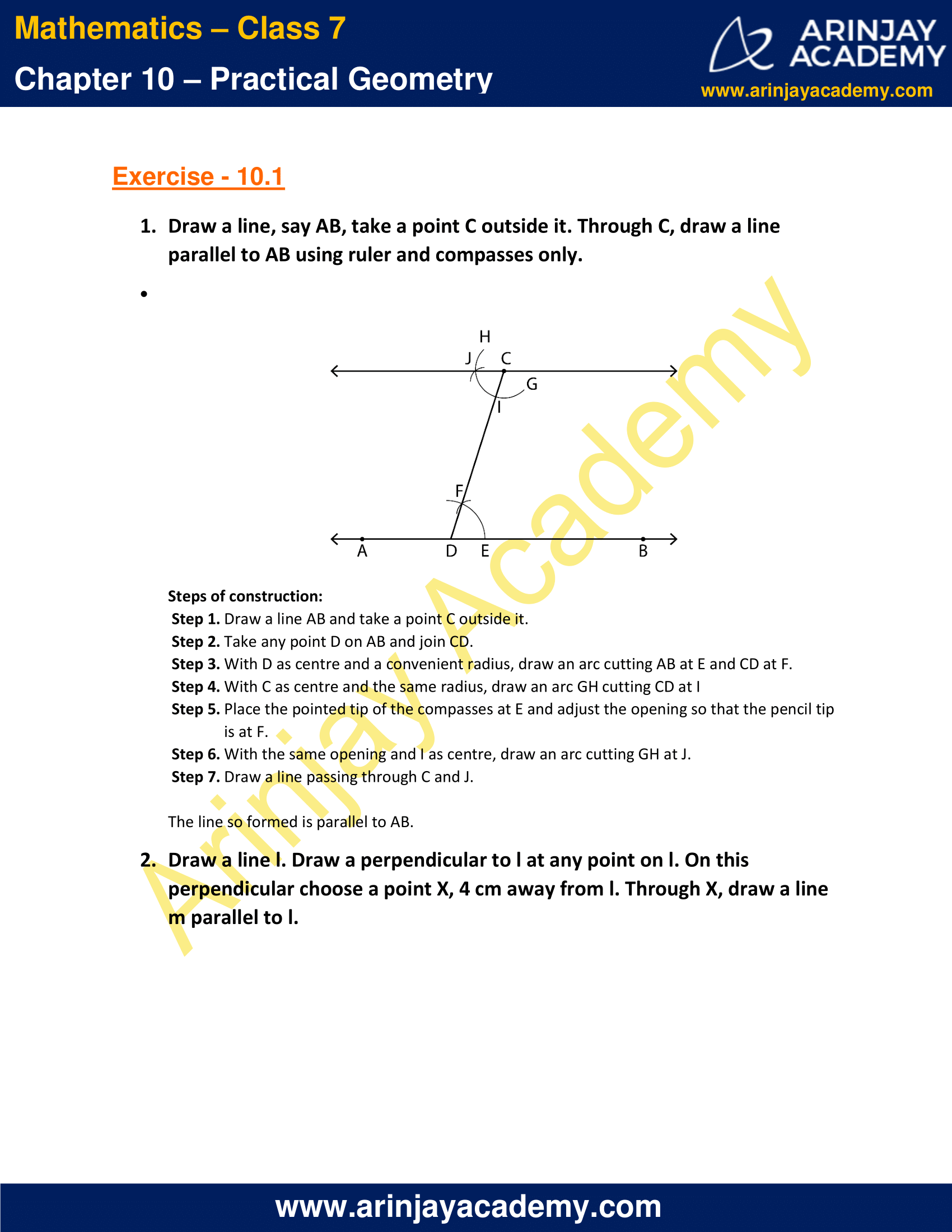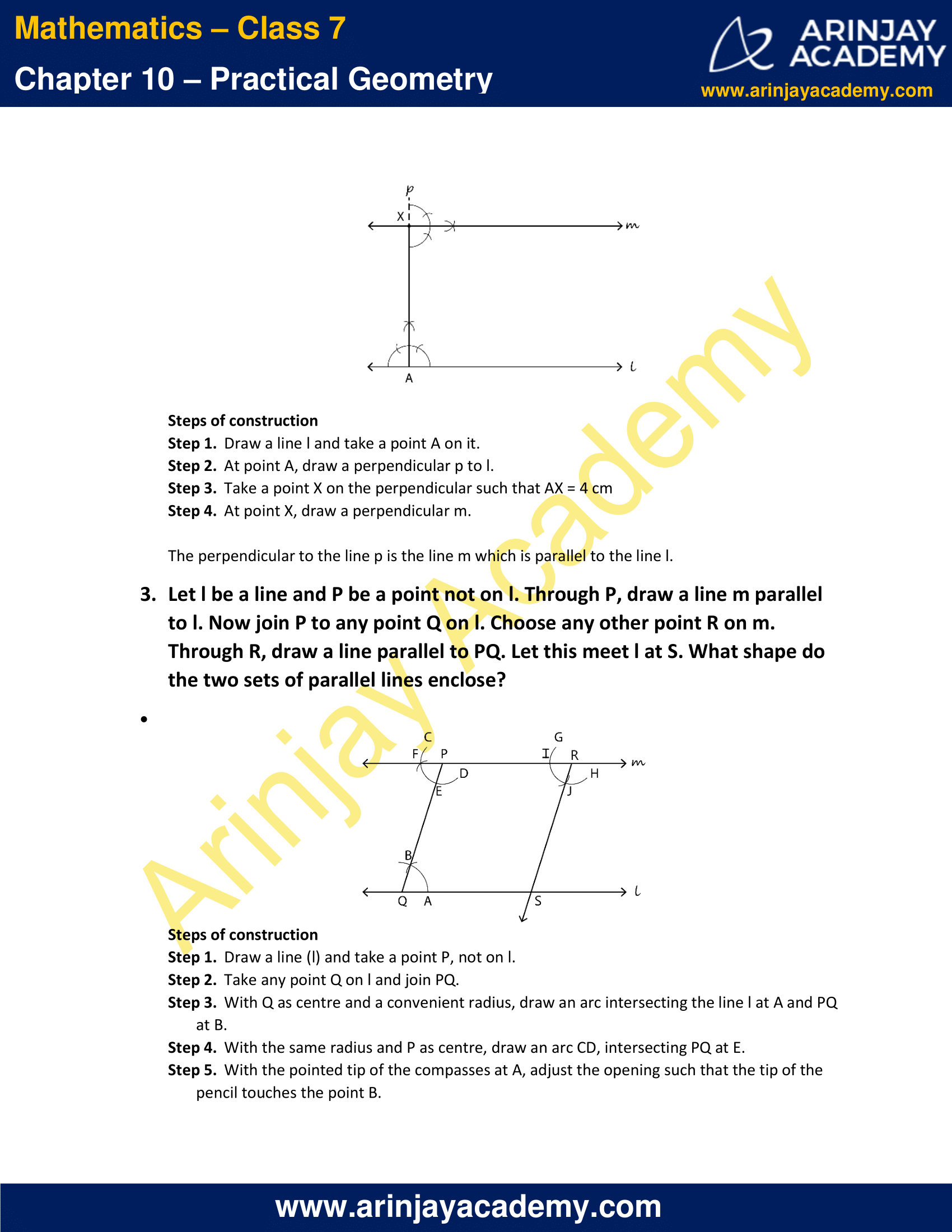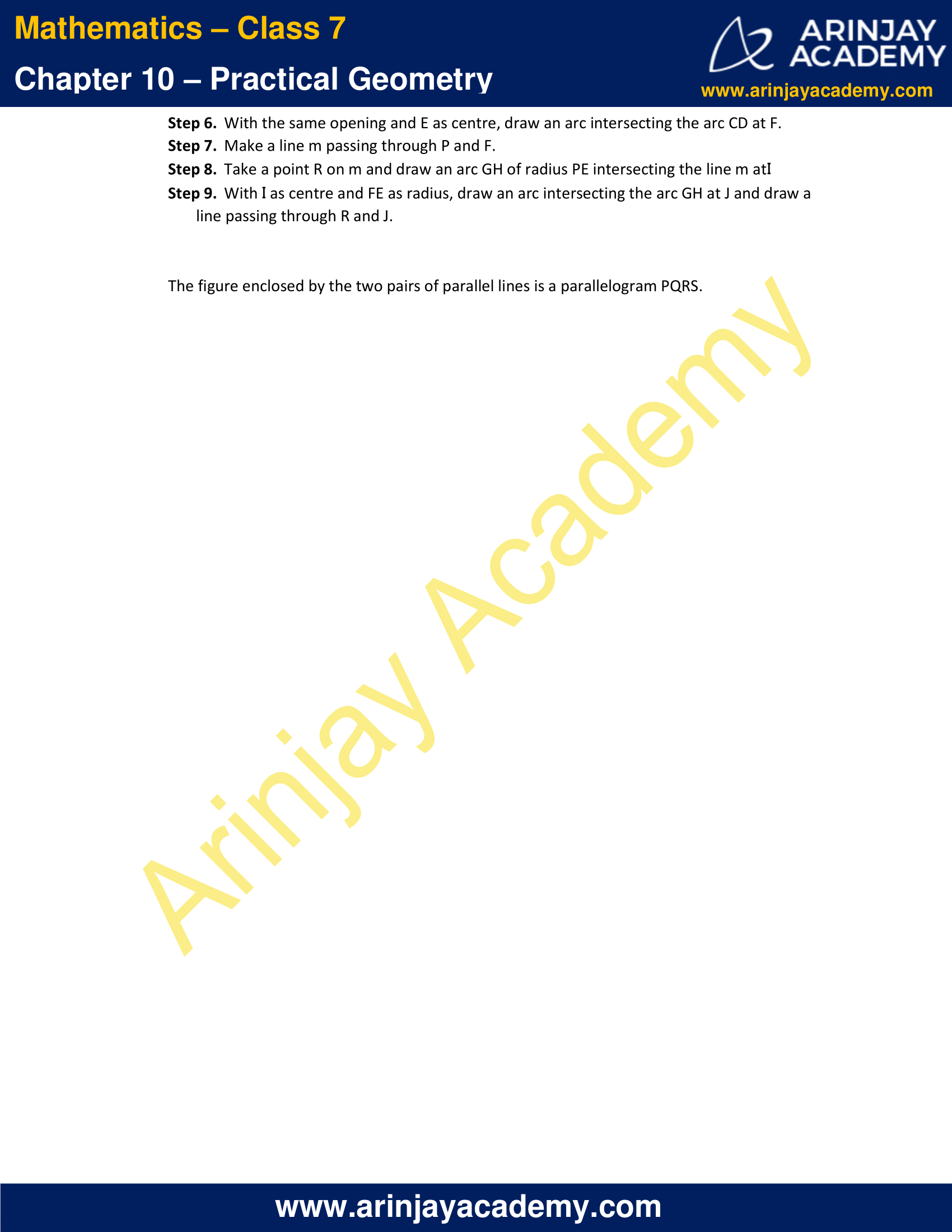### NCERT Solutions for Class 7 Maths Chapter 10 Exercise 10.2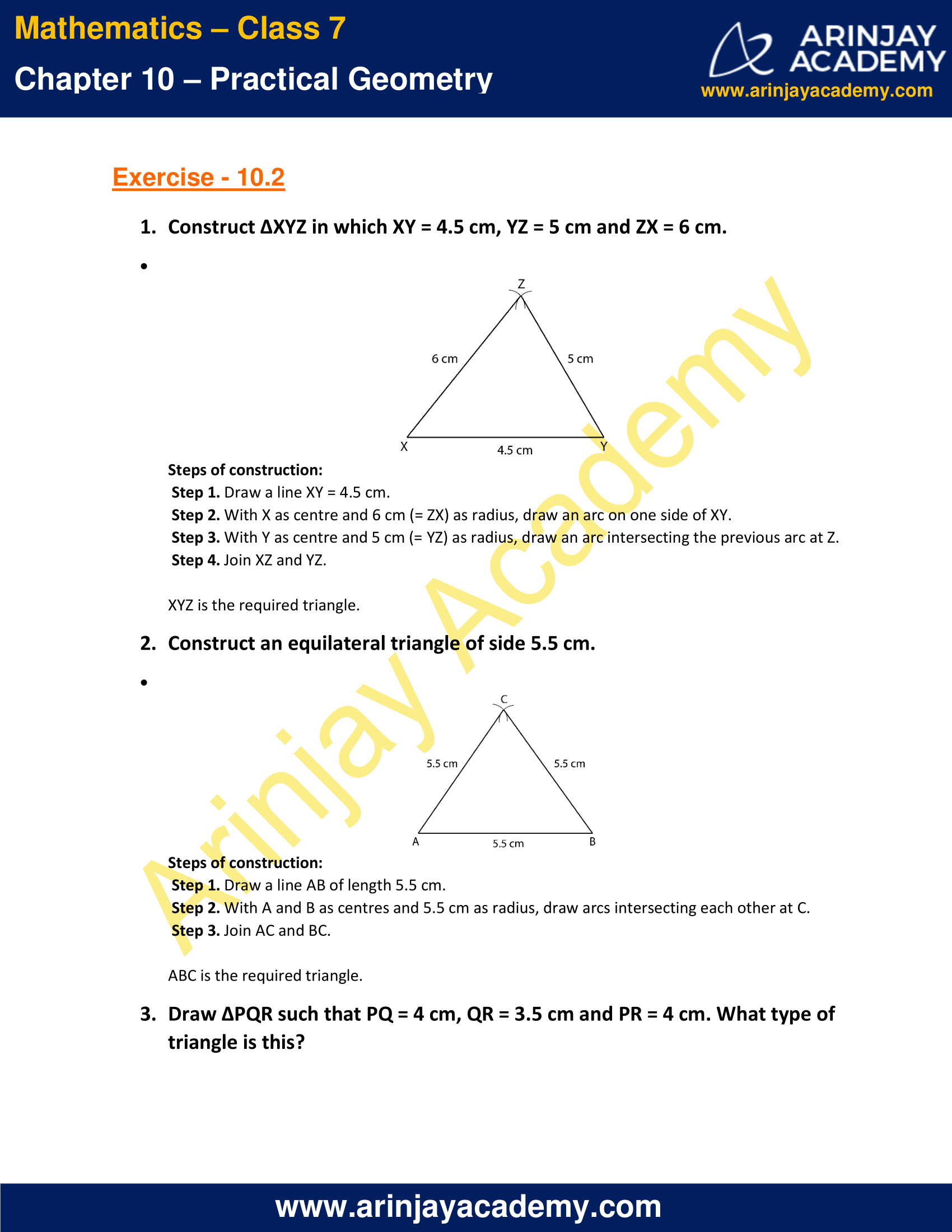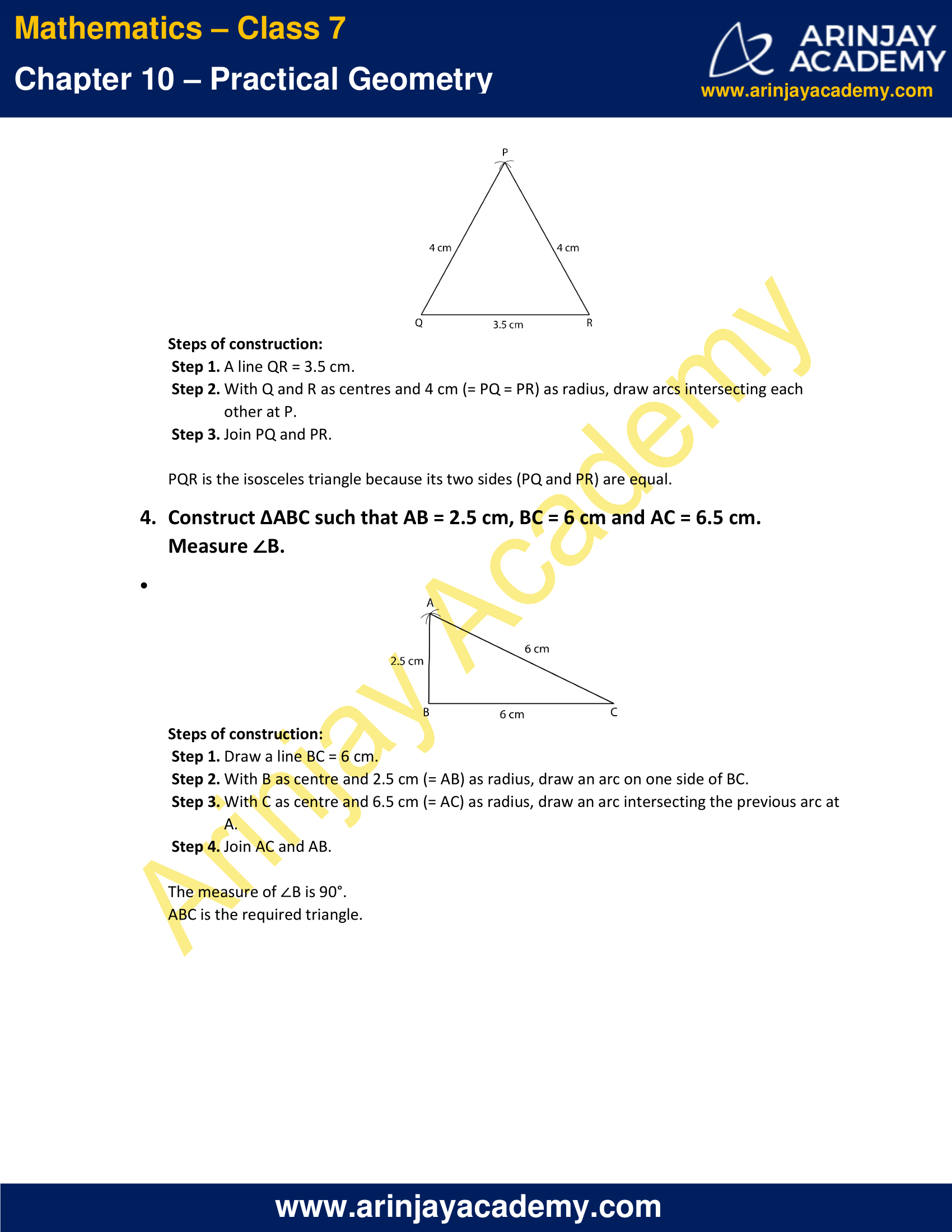### NCERT Solutions for Class 7 Maths Chapter 10 Exercise 10.3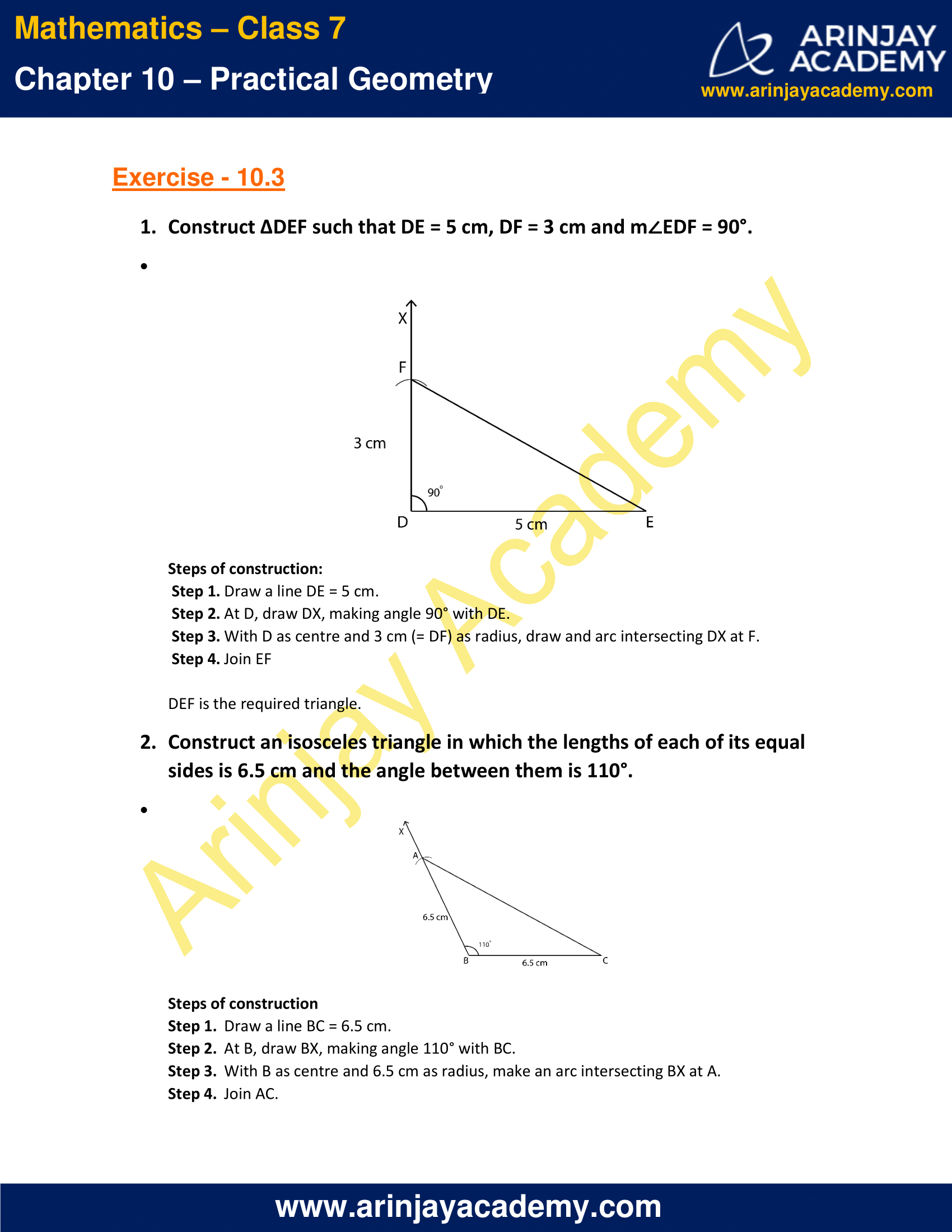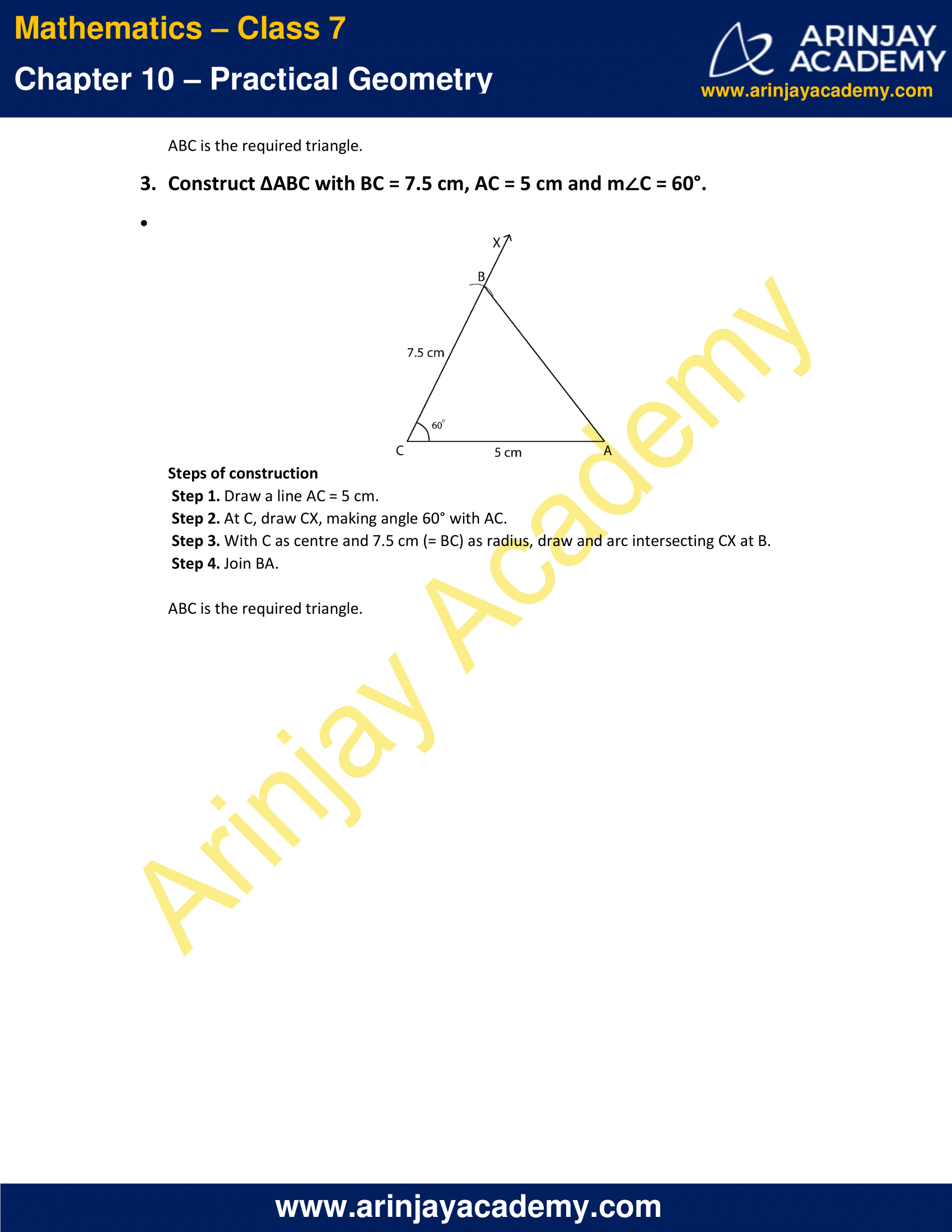### NCERT Solutions for Class 7 Maths Chapter 10 Exercise 10.4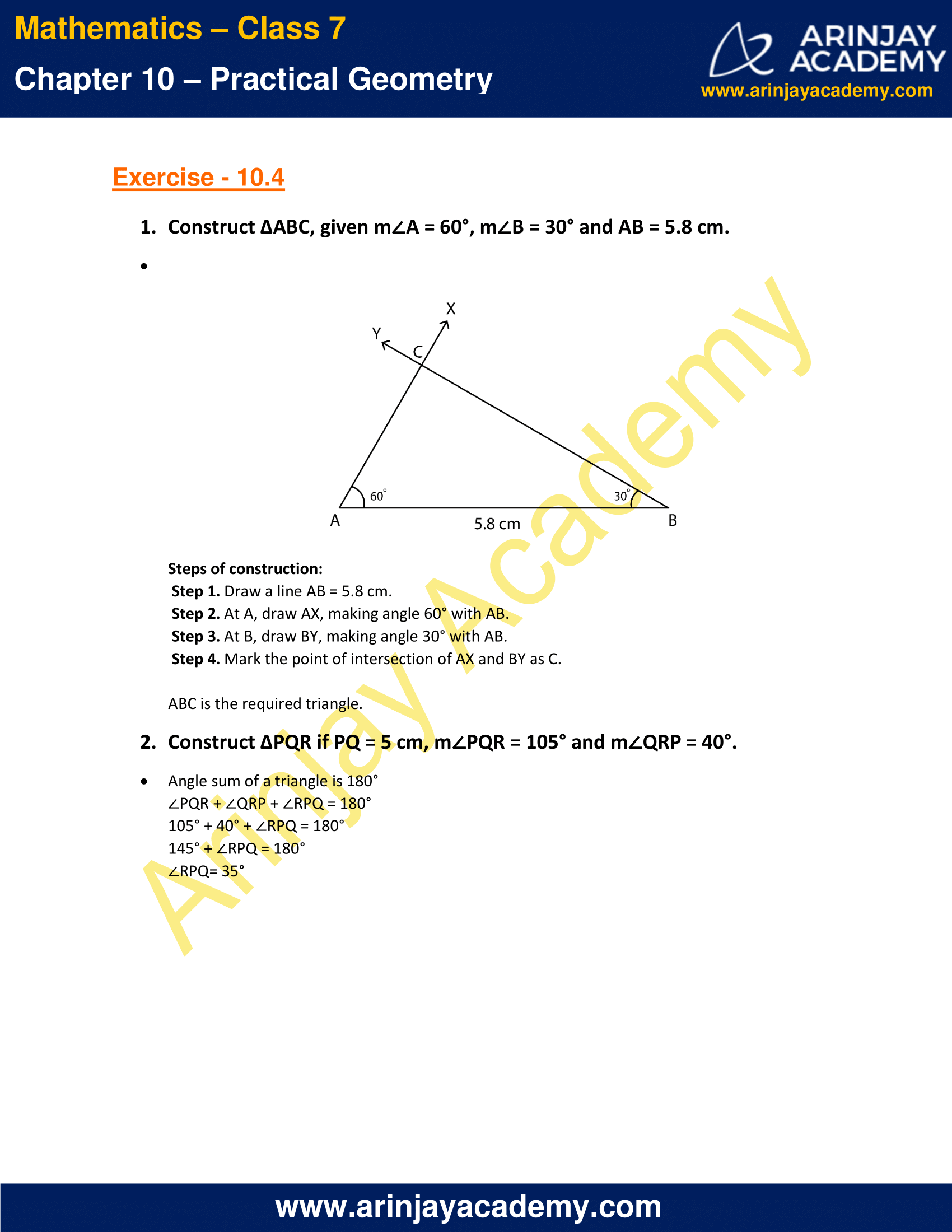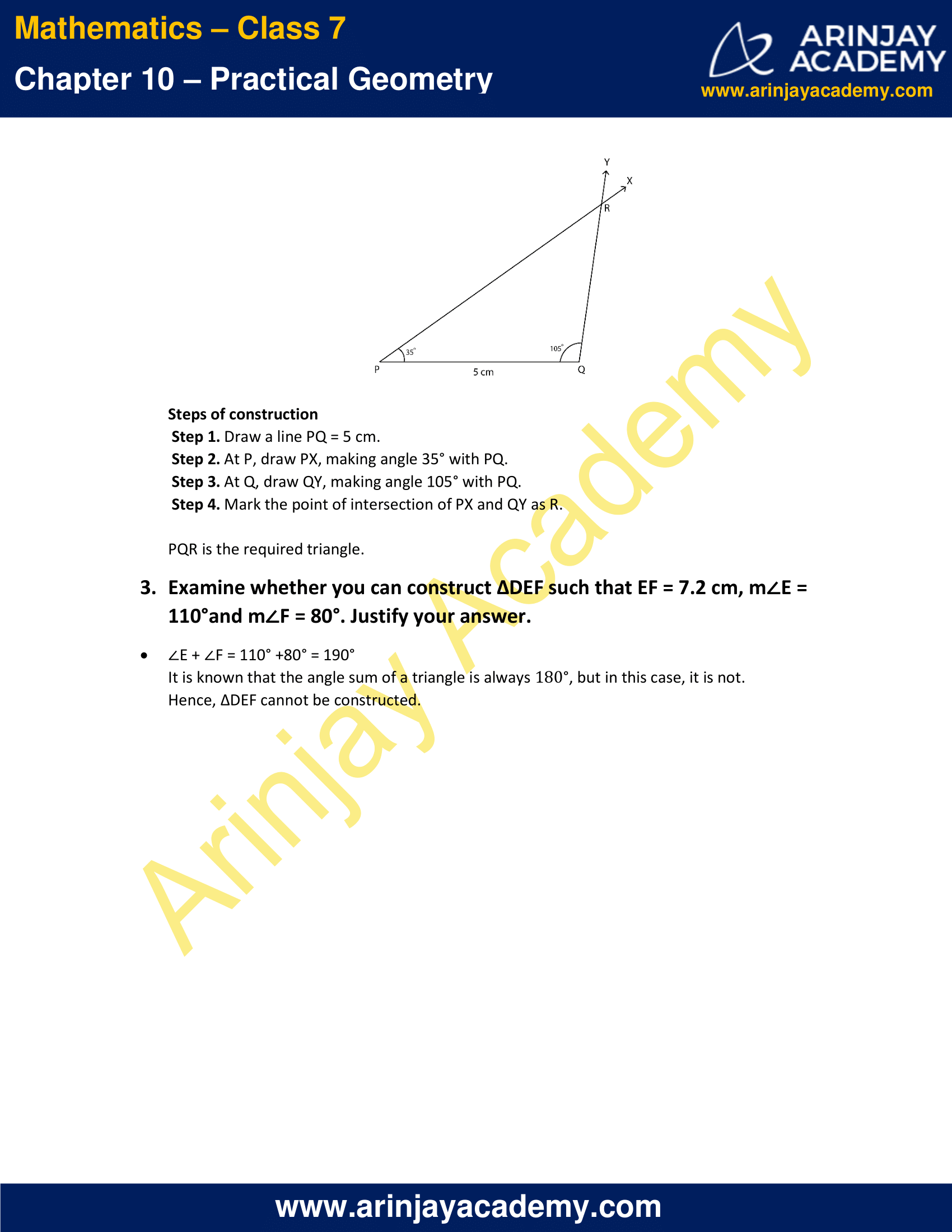### NCERT Solutions for Class 7 Maths Chapter 10 Exercise 10.5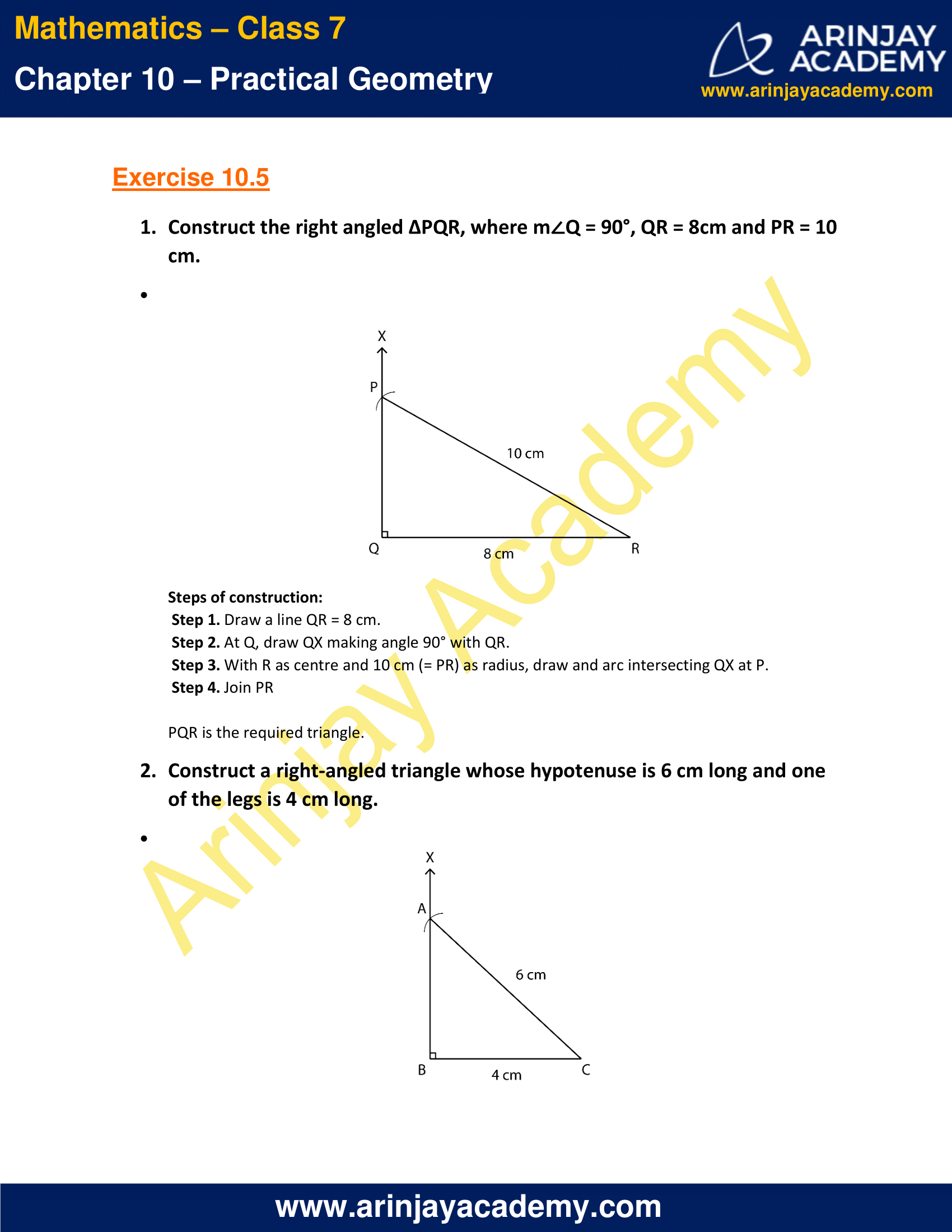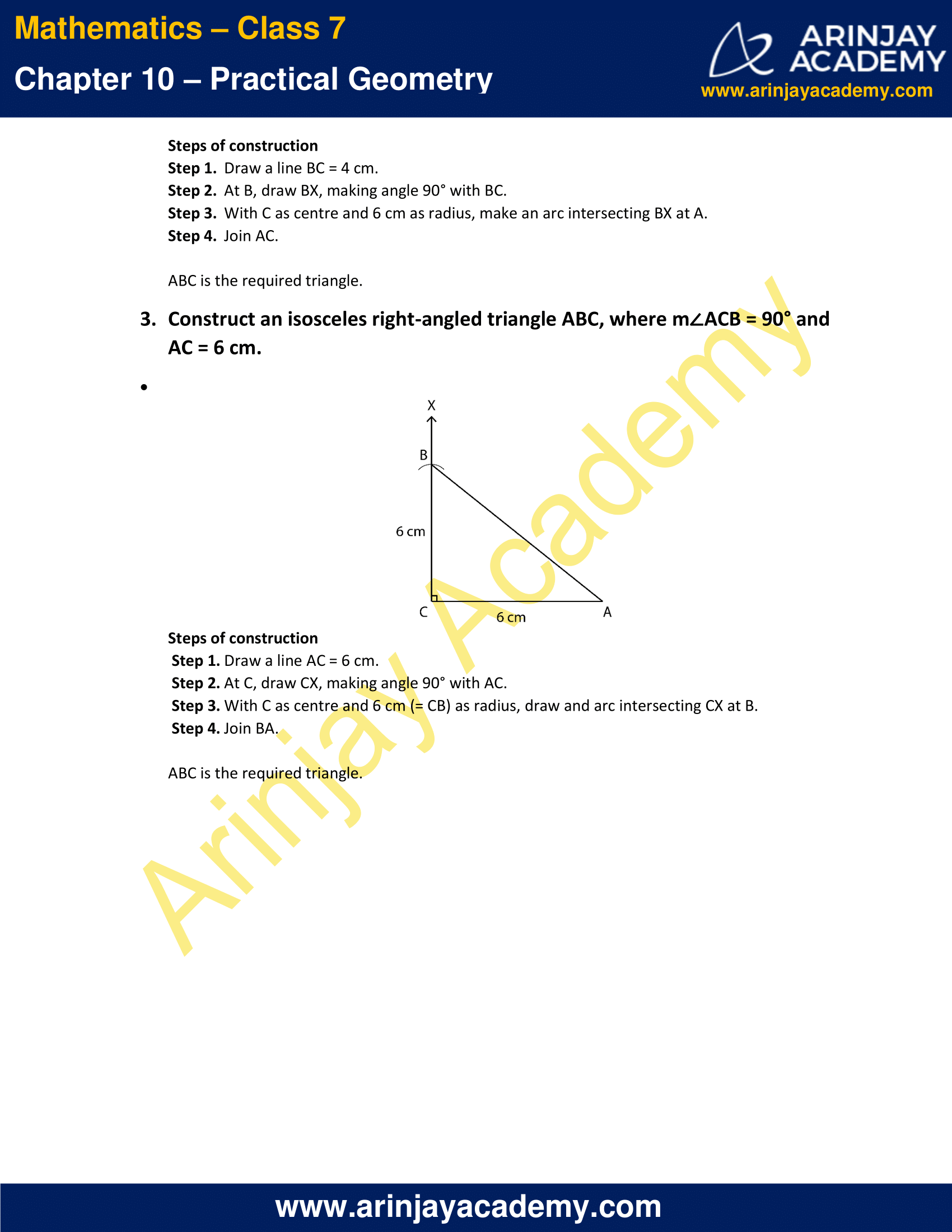### NCERT Solutions for Class 7 Maths Chapter 10 Exercise 10.1

1. Draw a line, say AB, take a point C outside it. Through C, draw a line parallel to AB using ruler and compasses only.

Solution: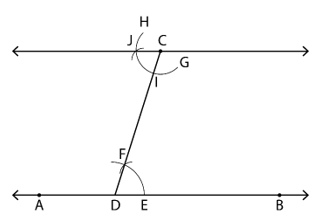Steps of construction:

• Step 1. Draw a line AB and take a point C outside it.
• Step 2. Take any point D on AB and join CD.
• Step 3. With D as centre and a convenient radius, draw an arc cutting AB at E and CD at F.
• Step 4. With C as centre and the same radius, draw an arc GH cutting CD at I
• Step 5. Place the pointed tip of the compasses at E and adjust the opening so that the pencil tip is at F.
• Step 6. With the same opening and I as centre, draw an arc cutting GH at J.
• Step 7. Draw a line passing through C and J.

The line so formed is parallel to AB.

2. Draw a line l. Draw a perpendicular to l at any point on l. On this perpendicular choose a point X, 4 cm away from l. Through X, draw a line m parallel to l.

Solution: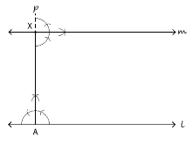Steps of construction

• Draw a line l and take a point A on it.
• At point A, draw a perpendicular p to l.
• Take a point X on the perpendicular such that AX = 4 cm
• At point X, draw a perpendicular m.

The perpendicular to the line p is the line m which is parallel to the line l.

3. Let l be a line and P be a point not on l. Through P, draw a line m parallel to l. Now join P to any point Q on l. Choose any other point R on m. Through R, draw a line parallel to PQ. Let this meet l at S. What shape do the two sets of parallel lines enclose?

Solution:Steps of construction

• Draw a line (l) and take a point P, not on l.
• Take any point Q on l and join PQ.
• With Q as centre and a convenient radius, draw an arc intersecting the line l at A and PQ at B.
• With the same radius and P as centre, draw an arc CD, intersecting PQ at E.
• With the pointed tip of the compasses at A, adjust the opening such that the tip of the pencil touches the point B.
• With the same opening and E as centre, draw an arc intersecting the arc CD at F.
• Make a line m passing through P and F.
• Take a point R on m and draw an arc GH of radius PE intersecting the line m at I
• With I as centre and FE as radius, draw an arc intersecting the arc GH at J and draw a line passing through R and J.

The figure enclosed by the two pairs of parallel lines is a parallelogram PQRS.

### NCERT Solutions for Class 7 Maths Chapter 10 Exercise 10.2

1. Construct ∆XYZ in which XY = 4.5 cm, YZ = 5 cm and ZX = 6 cm.

Solution:Steps of construction:

• Step 1. Draw a line XY = 4.5 cm.
• Step 2. With X as centre and 6 cm (= ZX) as radius, draw an arc on one side of XY.
• Step 3. With Y as centre and 5 cm (= YZ) as radius, draw an arc intersecting the previous arc at Z.
• Step 4. Join XZ and YZ.

XYZ is the required triangle.

2. Construct an equilateral triangle of side 5.5 cm.

Solution:Steps of construction:

• Step 1. Draw a line AB of length 5.5 cm.
• Step 2. With A and B as centres and 5.5 cm as radius, draw arcs intersecting each other at C.
• Step 3. Join AC and BC.

ABC is the required triangle.

3. Draw ∆PQR such that PQ = 4 cm, QR = 3.5 cm and PR = 4 cm. What type of triangle is this?

Solution:Steps of construction:

• Step 1. A line QR = 3.5 cm.
• Step 2. With Q and R as centres and 4 cm (= PQ = PR) as radius, draw arcs intersecting each other at P.
• Step 3. Join PQ and PR.

PQR is the isosceles triangle because its two sides (PQ and PR) are equal.

4. Construct ∆ABC such that AB = 2.5 cm, BC = 6 cm and AC = 6.5 cm. Measure ∠B

Solution:Steps of construction:

• Step 1. Draw a line BC = 6 cm.
• Step 2. With B as centre and 2.5 cm (= AB) as radius, draw an arc on one side of BC.
• Step 3. With C as centre and 6.5 cm (= AC) as radius, draw an arc intersecting the previous arc at A.
• Step 4. Join AC and AB.

The measure of ∠B is 90°.
ABC is the required triangle.

### NCERT Solutions for Class 7 Maths Chapter 10 Exercise 10.3

1. Construct ∆DEF such that DE = 5 cm, DF = 3 cm and m∠EDF = 90°.

Solution:Steps of construction:

• Step 1. Draw a line DE = 5 cm.
• Step 2. At D, draw DX, making angle 90° with DE.
• Step 3. With D as centre and 3 cm (= DF) as radius, draw and arc intersecting DX at F.
• Step 4. Join EF

DEF is the required triangle.

2. Construct an isosceles triangle in which the lengths of each of its equal sides is 6.5 cm and the angle between them is 110°.

Solution:Steps of construction

• Draw a line BC = 6.5 cm.
• At B, draw BX, making angle 110° with BC.
• With B as centre and 6.5 cm as radius, make an arc intersecting BX at A.
• Join AC.

ABC is the required triangle.

3. Construct ∆ABC with BC = 7.5 cm, AC = 5 cm and m∠C = 60°.

Solution:Steps of construction

• Step 1. Draw a line AC = 5 cm.
• Step 2. At C, draw CX, making angle 60° with AC.
• Step 3. With C as centre and 7.5 cm (= BC) as radius, draw and arc intersecting CX at B.
• Step 4. Join BA.

ABC is the required triangle.

### NCERT Solutions for Class 7 Maths Chapter 10 Exercise 10.4

1. Construct ∆ABC, given m∠A = 60°, m∠B = 30° and AB = 5.8 cm.

Solution:Steps of construction:

• Step 1. Draw a line AB = 5.8 cm.
• Step 2. At A, draw AX, making angle 60° with AB.
• Step 3. At B, draw BY, making angle 30° with AB.
• Step 4. Mark the point of intersection of AX and BY as C.

ABC is the required triangle.

2. Construct ∆PQR if PQ = 5 cm, m∠PQR = 105° and m∠QRP = 40°.

Solution:

Angle sum of a triangle is 180°
∠PQR + ∠QRP + ∠RPQ = 180°
105° + 40° + ∠RPQ = 180°
145° + ∠RPQ = 180°
∠RPQ = 35°Steps of construction

• Step 1. Draw a line PQ = 5 cm.
• Step 2. At P, draw PX, making angle 35° with PQ.
• Step 3. At Q, draw QY, making angle 105° with PQ.
• Step 4. Mark the point of intersection of PX and QY as R.

PQR is the required triangle.

3. Examine whether you can construct ∆DEF such that EF = 7.2 cm, m∠E = 110° and m∠F = 80°. Justify your answer.

Solution:

∠E + ∠F = 110° + 80° = 190°
It is known that the angle sum of a triangle is always 180°, but in this case, it is not.
Hence, ∆DEF cannot be constructed.

### NCERT Solutions for Class 7 Maths Chapter 10 Exercise 10.5

1. Construct the right angled ∆PQR, where m∠Q = 90°, QR = 8cm and PR = 10 cm.

Solution:Steps of construction:

• Step 1. Draw a line QR = 8 cm.
• Step 2. At Q, draw QX making angle 90° with QR.
• Step 3. With R as centre and 10 cm (= PR) as radius, draw and arc intersecting QX at P.
• Step 4. Join PR

PQR is the required triangle.

2. Construct a right-angled triangle whose hypotenuse is 6 cm long and one of the legs is 4 cm long.

Solution:Steps of construction

• Step 1 – Draw a line BC = 4 cm.
• Step 2 – At B, draw BX, making angle 90° with BC.
• Step 3 – With C as centre and 6 cm as radius, make an arc intersecting BX at A.
• Step 4 – Join AC.

ABC is the required triangle.

3. Construct an isosceles right-angled triangle ABC, where m∠ACB = 90° and AC = 6 cm.

Solution:Steps of construction

• Step 1. Draw a line AC = 6 cm.
• Step 2. At C, draw CX, making angle 90° with AC.
• Step 3. With C as centre and 6 cm (= CB) as radius, draw and arc intersecting CX at B.
• Step 4. Join BA.

ABC is the required triangle.

With this we come to the end of NCERT Solutions for Class 7 Maths Chapter 10 Practical Geometry. We hope these helped you study your subject.

Download NCERT Solutions for Class 7 Maths Chapter 10 – Practical Geometry